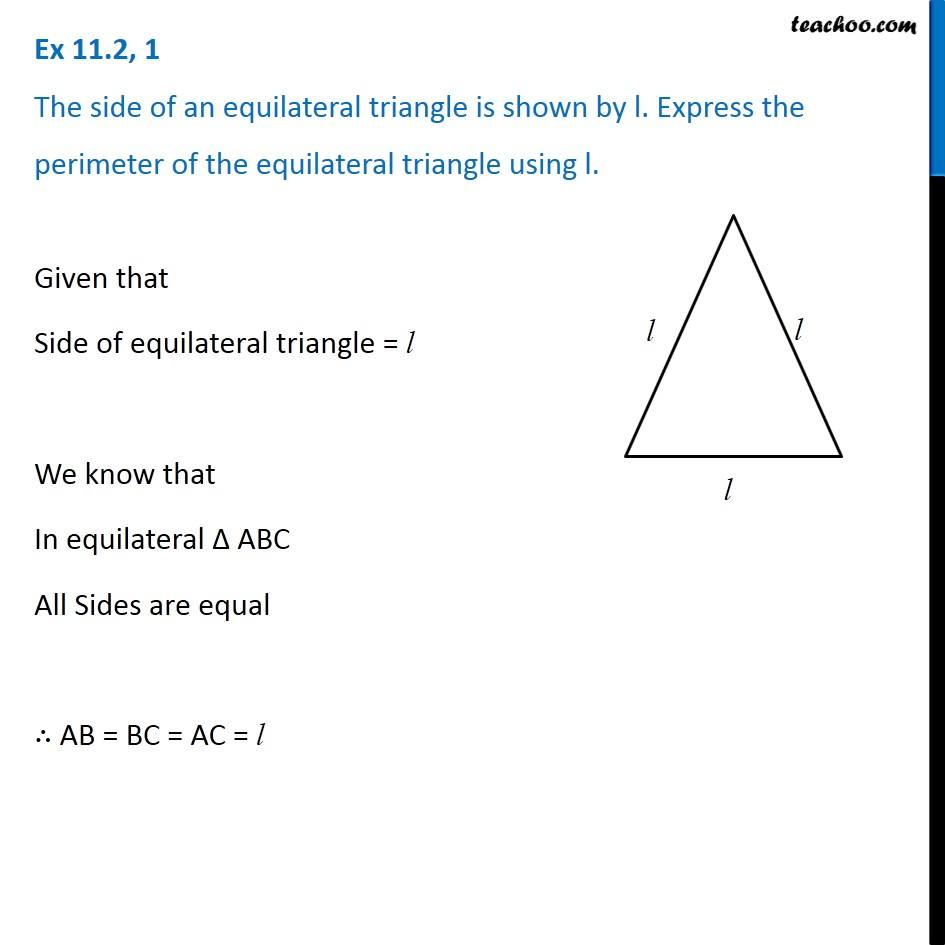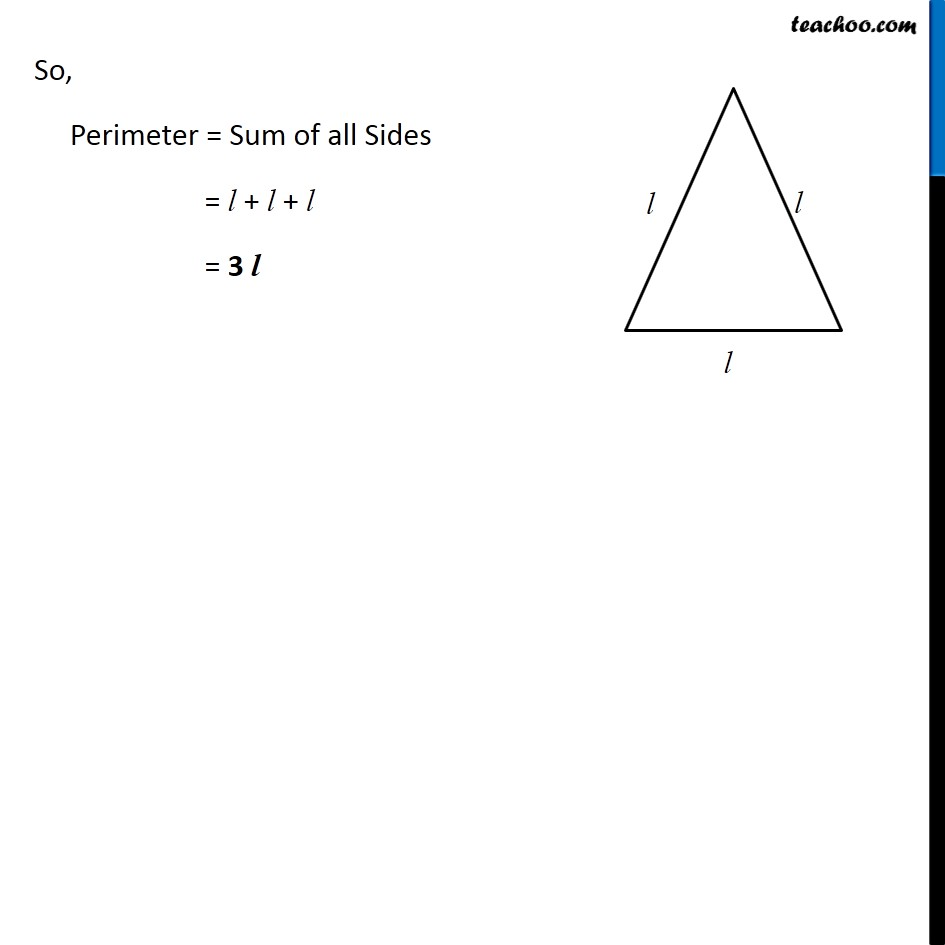Subscribe to our Youtube Channel - https://you.tube/teachoo

1. Chapter 11 Class 6 Algebra
2. Concept wise
3. Perimeter as variable

Transcript

Ex 11.2, 1 The side of an equilateral triangle is shown by l. Express the perimeter of the equilateral triangle using l. Given that Side of equilateral triangle = l We know that In equilateral ∆ ABC All Sides are equal ∴ AB = BC = AC = l So, Perimeter = Sum of all Sides = l + l + l = 3 l

Perimeter as variable